## Hermite Differential Equation(1)

This differential equation has an irregular singularity at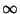. It can be solved using the series method(2)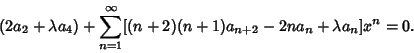(3)

Therefore,(4)

and(5)

for, 2, .... Since (4) is just a special case of (5),(6)

for, 1, .... The linearly independent solutions are then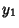(7)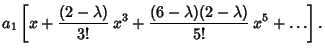(8)

If, 4, 8, ..., thenterminates with the Power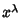, and(normalized so that the Coefficient ofis) is the regular solution to the equation, known as the Hermite Polynomial. If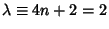, 6, 10, ..., then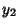terminates with the Power, and(normalized so that the Coefficient ofis) is the regular solution to the equation, known as the Hermite Polynomial.

If, then Hermite's differential equation becomes(9)

which is of the formand so has solution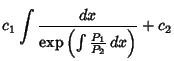(10)